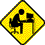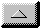Guest Editorial

Testing the Null Hypothesis
by John Marcus, MD
email MarcusJohn @ aol.com

SETI is perhaps the most highly interdisciplinary of sciences, encompassing not only astronomy, biology, engineering and physics, but also psychology, metaphysics, probability, and belief. But it is, first and foremost, a science, one to which we hope to apply the scientific method.

A colleague on the SETI email discussion list wrote, "scientists (and really, everybody, either consciously or unconsciously) use the scientific method for problem solving. It's just that most people don't realize that they are doing it!" Would that this were so. As a scientist, a mathematician, a physician, and a generally philosophical person, I like to think I know a little bit about problem solving and logic. And I think that the scientific method (proving the null hypothesis false) is rarely used in general problem solving. Lets take for instance the need to buy a car.

Scientific method: The null hypothesis is 'I cannot afford the car'. Now let me set about proving this null hypothesis void. I will first examine my bank account and my income....

Usual method: I probably can afford the car. Now let me see what this probability really is by examining my bank account and income....

The difference is subtle, but important. You can see that the scientific method involves a boolean logic variable of false, that once proven incorrect, leaves a boolean logic variable of true. Once something has been proven true, you have added new information to the sum of human knowledge. But in real life we usually deal with lack of certainty. So we have probabilities, instead of boolean logic. This is a different kind of math. This math was described by a person named Bayes. And there is a Bayes theorem for this kind of logic. Bayes theorem goes like this:

There is an a-priori probability (a number between 0 and 1).
There is an a-posteriori probability (a number between 0 and 1).
There is a test, which examines reality, and changes the probability.

post-p = test (prior-p)
You can see that the above involves a mathematical function. This function may be found on the web.

Let's work a sample problem. I believe that there is a 30 percent chance that I can afford that turbo 911 Carrera. I want to examine this probabilily. (This is the problem solving goal). Now I will peform the test: look at my bank account and income. There is not enough there. Oh well, now there is an a-posteriori probability of 10 percent chance of me affording that Carrera. Now I have another test: I will discuss it with my wife. After careful deliberation, she says no. Well, that lowers the probability to about 1 percent. The probability is not zero, because I could do some things that would force the issue, and raise the probability. But as things stand right now, it remains unlikely that I will buy a Carrera.

A web search on Bayes Theorem will turn up a ton of references. I leave them as an exercise for the student.

Let's see how the above applies to SETI. Do I have belief in ET's? No, but I have a probability in mind. You may wish to phrase the question differently: do I have a belief in the probability of ET's? Yes I do. I wish to apply a test (the Argus search). There are other tests. These may raise the probability, if the search succeeds.

The Scientific Method for the Argus search is this:

There are no ET's. (null hypothesis).
You can never prove the negative. So we now design an experiment (Project Argus, for example) to try to prove that statement wrong, recognizing that it takes only one clear, unambiguous counter-example to reject the null hypothesis.

I find the scientific method to be artificial and limiting, because in real life we never deal with any certainty about anything. On the other hand, if it is certainty which we seek, then the scientific method is probably the best way to achieve it.email theWebmaster | Home | General | Memb Svcs | Publications | Press | Technical | Internet | Index | entire website copyright © The SETI League, Inc. this page last updated 4 January 2003Top of Page